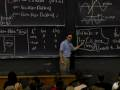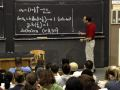# Session 25: Introduction to Quadratic Approximation« Previous | Next »

## Overview

Linear approximation uses the first derivative to find the straight line that most closely resembles a curve at some point. Quadratic approximation uses the first and second derivatives to find the parabola closest to the curve near a point.

## Lecture Video and Notes

### Video Excerpts

Flash and JavaScript are required for this feature.From Lecture 9 of 18.01 Single Variable Calculus, Fall 2006

Flash and JavaScript are required for this feature.» Clip 2: Explaining the Formula by Example (00:04:00)

From Lecture 10 of 18.01 Single Variable Calculus, Fall 2006

Flash and JavaScript are required for this feature.From Lecture 9 of 18.01 Single Variable Calculus, Fall 2006

## Worked Example

Comparing Quadratic Approximations and Calculator Computations

## Recitation Video

### Quadratic Approximation of an Exponential Function

Flash and JavaScript are required for this feature.

« Previous | Next »